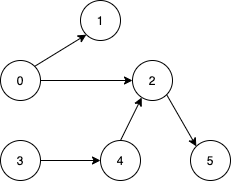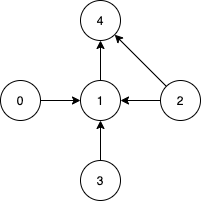1557. Minimum Number of Vertices to Reach All Nodes

#### QUESTION:

Given a directed acyclic graph, with n vertices numbered from 0 to n-1, and an array edges where edges[i] = [fromi, toi] represents a directed edge from node fromi to node toi.

Find the smallest set of vertices from which all nodes in the graph are reachable. It’s guaranteed that a unique solution exists.

Notice that you can return the vertices in any order.

Example 1:``````Input: n = 6, edges = [[0,1],[0,2],[2,5],[3,4],[4,2]]
Output: [0,3]
Explanation: It's not possible to reach all the nodes from a single vertex. From 0 we can reach [0,1,2,5]. From 3 we can reach [3,4,2,5]. So we output [0,3].
``````

Example 2:``````Input: n = 5, edges = [[0,1],[2,1],[3,1],[1,4],[2,4]]
Output: [0,2,3]
Explanation: Notice that vertices 0, 3 and 2 are not reachable from any other node, so we must include them. Also any of these vertices can reach nodes 1 and 4.
``````

Constraints:

``````2 <= n <= 10^5
1 <= edges.length <= min(10^5, n * (n - 1) / 2)
edges[i].length == 2
0 <= fromi, toi < n
All pairs (fromi, toi) are distinct.
``````

#### EXPLANATION:

1.首先for循环取出edges[i],拿到被指向的数
2.把这个被指向的数作为index,在bool array里标记为有指向
3.遍历bool array, 将没有被指向的index放到结果中

#### SOLUTION:

``````class Solution {
func findSmallestSetOfVertices(_ n: Int, _ edges: [[Int]]) -> [Int] {
var arr:[Bool] = Array(repeating: false, count: n)
var result:[Int] = []
for edge in edges {
arr[edge] = true
}
for index in arr.indices {
if (!arr[index]) {
result.append(index)
}
}
return result
}
}
``````